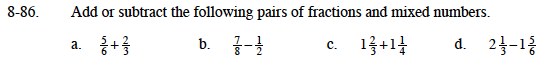### Home > MC1 > Chapter 8 > Lesson 8.3.1 > Problem8-86

8-86.$\frac{5}{6}+\frac{4}{6}$

Which Giant One should you use to make both numbers have 8 in the denominator?

$\frac{5}{3}+\frac{5}{4}$

$\frac{1}{2}$

Can you show why this makes sense with a diagram?